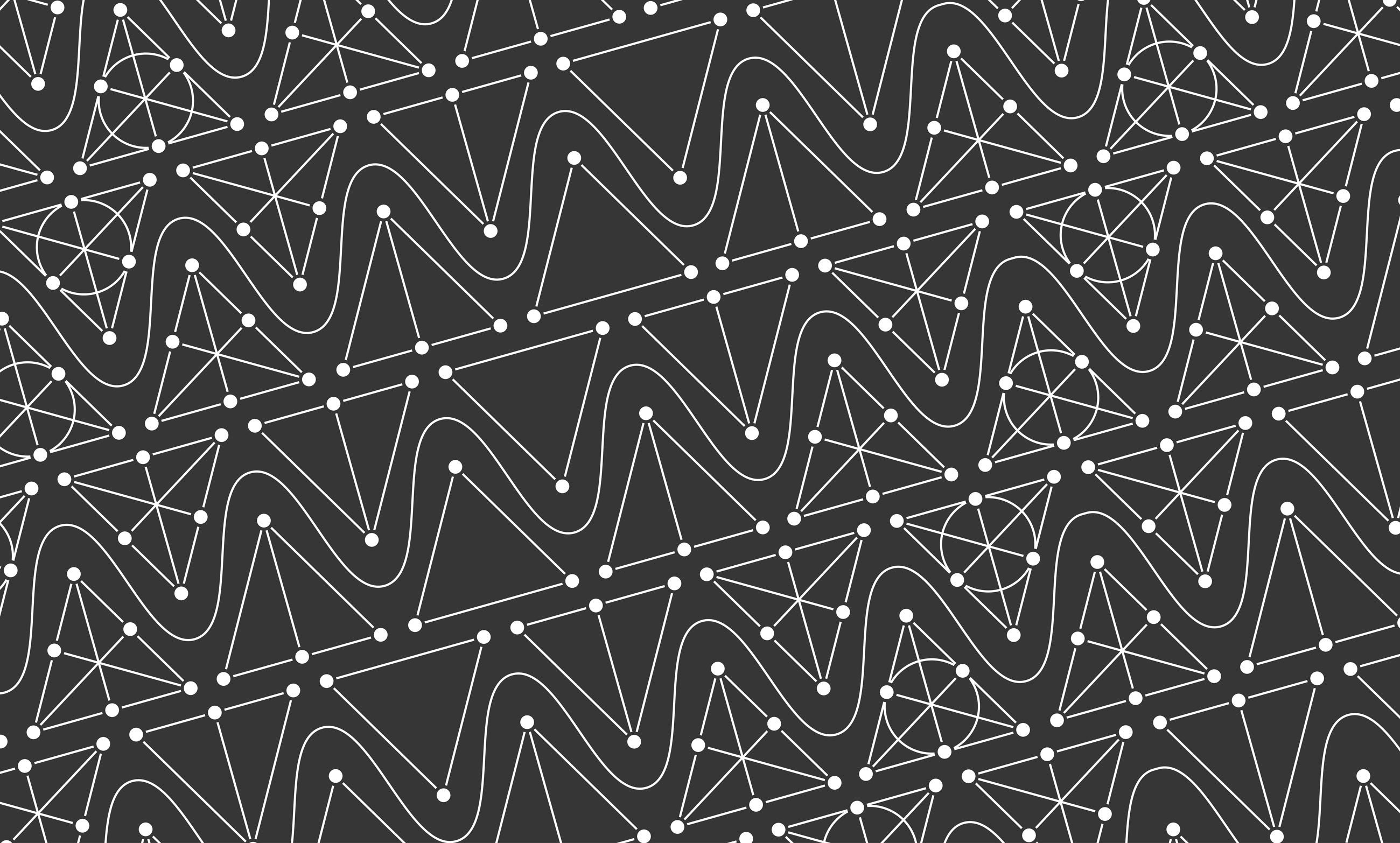# The Tricky Translation of Mathematical Ideas

Big advances in math can happen when mathematicians move ideas into areas where they seem like they shouldn’t belong.Explore

My latest story is about the mathematician June Huh, who came into the field late and by an unorthodox path. Huh’s approach to mathematics has been similarly surprising: He and his two collaborators, Eric Katz and Karim Adiprasito, solved an important problem called the Rota conjecture by figuring out how to translate ideas from one area of math to a realm where those ideas wouldn’t seem to belong.

Breakthroughs in mathematics often come by surprising means—problems that seemed hopeless become solvable when mathematicians find a new interpretation for established ideas. This means more than finding a new use for an old tool. It’s really about extending a pattern of argument that arose in one setting into another.

A good example of how this kind of translation works involves a concept called the Laplacian. The original Laplacian was developed by the 18th-century scholar Pierre-Simon Laplace to understand planetary motion and is constructed out of the second derivatives of a function. That function, for example, might describe Jupiter’s trajectory in space, in which case the Laplacian of the function would tell you something about its gravitational energy at a specific time and place.

The Laplacian arose in the world of continuous mathematics, where functions describe smooth, unbroken spaces. In discrete mathematics, by contrast, you have objects like graphs, which are collections of points (vertices) glued together by sticks (edges). Yet there is such a thing as the “graph Laplacian,” which follows from certain non-obvious properties of the original Laplacian. It’s not really a derivative, and it’s not calculated in the same way as Laplace’s Laplacian, but it retains its spirit.

To calculate the graph Laplacian, first imagine a graph and also a function on that graph that outputs a value at each point. For example, maybe the points of the graph represent discrete points in Jupiter’s orbit, which can be input into a function that tells you Jupiter’s velocity at those points. The Laplacian of that function is a new function, which assigns new values to each point (just like the derivative or second derivative of a function assigns new values to each input).

Here’s how you calculate it. Say point A is connected to points B and C. To calculate the value of the Laplacian at A, first add the values of the function at neighboring points, then subtract the value at A times the number of ways A is connected to other points.

On a graph, subtracting the values of connected points is the appropriate analog of taking a derivative, which is, after all, the difference of two values infinitely close together. And given that, differentiating a function becomes like taking the difference between two points connected by an edge. (For a particular reason related to something called Green’s second identity, this subtraction operation mirrors the second derivative, rather than the first.)

The correct analogue of an established mathematical idea is often not obvious, and finding it can take awhile. But once established, it becomes quite powerful. Huh, Katz, and Adiprasito spent four years searching for the right way to translate certain ideas into the realm of graphs. Once they’d found it, a proof of the Rota conjecture followed in a matter of a few months.Enjoy unlimited Nautilus articles, ad-free, for as little as \$4.92/month.